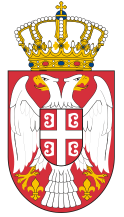Dokument se učitava

# Computer Architecture and Organization ICourse code: 130107 | 6 ECTS credits

Basic information
Level of Studies: Undergraduate applied studies
Year of Study: 1
Semester: 1
Requirements: None
Goal: Acquiring basic knowledge of architecture, mathematical and electronic basics of computer systems.
Outcome: Possibility to independently solve tasks based on the application of binary arithmetic and basic logic circuits.
Contents of the course
Theoretical instruction:
1. General model of computer system. Functional computer block diagram. Hierarchical model of computer system.
2. Mathematical base of computer system. Numbering system. Conversion from one number system to another.
3. Binary number system. Signed and unsigned numbers. Fixed point and floating point presentation in computer memory.
4. Basic arithmetic operations in binary number system.
5. Numeric and nonnumeric data presentation using binary codes.
6. Basic computer electronics. Axioms of Boolean algebra. Basic logic gates and logic circuits.
7. Logic functions. Simplification using Karnaugh maps.
8. Basic combinational logic networks: decoder, coder, multiplexer, demultiplexer, comparator, half adder, adder.
9. Basic memory elements: synchronous and asynchronous flip flops (RS, JK, D, T). Concept of sequence network. Synchronous and asynchronous counters.
10. Types of registers, depending on reading and writing methods. Special purpose registers.
11. Unary and binary operations with registry. Applying basic logic operations and mask register.
12. Basic computer systems components. Realization of control units. Micro program and hardware implementation. Arithmetic logic unit.
13. Memory in computer system. Properties of ROM, RAM and stack memories.
14. Simplified computer architecture. Data transfer. Memory access.
15. Computer peripherals. Input/output data transferring methods.
Practical instruction (Problem solving sessions/Lab work/Practical training):
1. Practical training program follows the lectures. Module is in compliance to IEEE/ACM Computing Curriculum: CE2004 Computer Engineering Body of Knowledge: CE-CAO 0-3
Textbooks and References
2. D. Prokin, M. Mijalković, V. Petrović, Zbirka zadataka iz Osnova računatske tehnike, VIŠER, Beograd, 2013.
3. D. Prokin, M. Mijalković, G. Dimić, B. Bogojević, P. Gavrilović, D. Mićić, Priručnik za laboratorijske vežbe iz Arhitekture i organizacije računara 1, VIŠER, Beograd, 2018.
Number of active classes (weekly)
Lectures: 3
Practical classes: 2
Other types of classes: 0
Grading (maximum number of points: 100)
Pre-exam obligations
Points
activities during lectures
5
activities on practial excersises
15
seminary work
10
colloquium
40
Final exam
Points
Written exam
30
Oral exam
0© 2019 School of Electrical and Computer Engineering of Applied Studies, Belgrade
Vojvode Stepe 283, office@viser.edu.rs, +381 11 2471 099